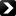Under the auspices of the Computational Complexity Foundation (CCF)LATEST > REPORTS:NextTR21-147 | 22nd October 2021

#### Extractors for Sum of Two Sources

Revisions: 1

We consider the problem of extracting randomness from \textit{sumset sources}, a general class of weak sources introduced by Chattopadhyay and Li (STOC, 2016). An $(n,k,C)$-sumset source $\mathbf{X}$ is a distribution on $\{0,1\}^n$ of the form $\mathbf{X}_1 + \mathbf{X}_2 + \ldots + \mathbf{X}_C$, where $\mathbf{X}_i$'s are independent sources on $n$ bits ... more >>>

TR21-146 | 19th October 2021
Guy Goldberg, Guy Rothblum

#### Sample-Based Proofs of Proximity

Suppose we have random sampling access to a huge object, such as a graph or a database.
Namely, we can observe the values of \emph{random} locations in the object, say random records in the database or random edges in the graph.
We cannot, however, query locations of our choice. Can ... more >>>

TR21-145 | 19th October 2021
Omar Alrabiah, Venkatesan Guruswami

#### Revisiting a Lower Bound on the Redundancy of Linear Batch Codes

A recent work of Li and Wootters (2021) shows a redundancy lower bound of $\Omega(\sqrt{Nk})$ for systematic linear $k$-batch codes of block length $N$ by looking at the $O(k)$ tensor power of the dual code. In this note, we present an alternate proof of their result via a linear independence ... more >>>

NextISSN 1433-8092 | Imprint